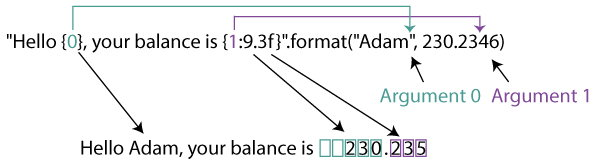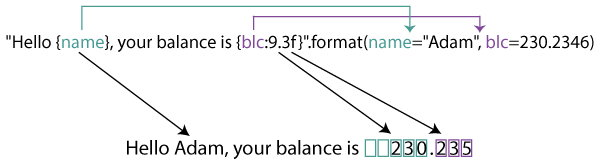# Python String format()

The string format() method formats the given string into a nicer output in Python.

The syntax of format() method is:

`template.format(p0, p1, ..., k0=v0, k1=v1, ...)`

Here, p0, p1,... are positional arguments and, k0, k1,... are keyword arguments with values v0, v1,... respectively.

And, template is a mixture of format codes with placeholders for the arguments.

## String format() Parameters

format() method takes any number of parameters. But, is divided into two types of parameters:

• Positional parameters - list of parameters that can be accessed with index of parameter inside curly braces {index}
• Keyword parameters - list of parameters of type key=value, that can be accessed with key of parameter inside curly braces {key}

## Return value from String format()

The format() method returns the formatted string.

## How String format() works?

The format() reads the type of arguments passed to it and formats it according to the format codes defined in the string.

### For positional argumentsHere, Argument 0 is a string "Adam" and Argument 1 is a floating number 230.2346.

Note: Argument list starts from 0 in Python.

The string `"Hello {0}, your balance is {1:9.3f}"` is the template string. This contains the format codes for formatting.

The curly braces are just placeholders for the arguments to be placed. In the above example, {0} is placeholder for "Adam" and {1:9.3f} is placeholder for 230.2346.

Since the template string references format() arguments as {0} and {1}, the arguments are positional arguments. They both can also be referenced without the numbers as {} and Python internally converts them to numbers.

Internally,

• Since "Adam" is the 0th argument, it is placed in place of {0}. Since, {0} doesn't contain any other format codes, it doesn't perform any other operations.
• However, it is not the case for 1st argument 230.2346. Here, `{1:9.3f}` places 230.2346 in its place and performs the operation 9.3f.
• f specifies the format is dealing with a float number. If not correctly specified, it will give out an error.
• The part before the "." (9) specifies the minimum width/padding the number (230.2346) can take. In this case, 230.2346 is allotted a minimum of 9 places including the ".".
If no alignment option is specified, it is aligned to the right of the remaining spaces. (For strings, it is aligned to the left.)
• The part after the "." (3) truncates the decimal part (2346) upto the given number. In this case, 2346 is truncated after 3 places.
Remaining numbers (46) is rounded off outputting 235.

### For keyword argumentsWe've used the same example from above to show the difference between keyword and positional arguments.

Here, instead of just the parameters, we've used a key-value for the parameters. Namely, name="Adam" and blc=230.2346.

Since, these parameters are referenced by their keys as {name} and {blc:9.3f}, they are known as keyword or named arguments.

Internally,

• The placeholder {name} is replaced by the value of name - "Adam". Since, it doesn't contain any other format codes, "Adam" is placed.
• For the argument blc=230.2346, the placeholder {blc:9.3f} is replaced by the value 230.2346. But before replacing it, like previous example, it performs 9.3f operation on it.
This outputs   230.235. The decimal part is truncated after 3 places and remaining digits are rounded off. Likewise, the total width is assigned 9 leaving two spaces to the left.

## Basic formatting with format()

The format() method allows the use of simple placeholders for formatting.

### Example 1: Basic formatting for default, positional and keyword arguments

```# default arguments

# positional arguments

# keyword arguments

# mixed arguments
```

When you run the program, the output will be same for all:

```Hello Adam, your balance is 230.2346.

Note: In case of mixed arguments, keyword arguments has to always follow positional arguments.

## Numbers formatting with format()

You can format numbers using the format specifier given below:

Number Formatting Types
Type Meaning
d Decimal integer
c Corresponding Unicode character
b Binary format
o Octal format
n Same as 'd'. Except it uses current locale setting for number separator
e Exponential notation. (lowercase e)
E Exponential notation (uppercase E)
f Displays fixed point number (Default: 6)
F Same as 'f'. Except displays 'inf' as 'INF' and 'nan' as 'NAN'
g General format. Rounds number to p significant digits. (Default precision: 6)
G Same as 'g'. Except switches to 'E' if the number is large.
% Percentage. Multiples by 100 and puts % at the end.

### Example 2: Simple number formatting

```# integer arguments
print("The number is:{:d}".format(123))

# float arguments
print("The float number is:{:f}".format(123.4567898))

# octal, binary and hexadecimal format
print("bin: {0:b}, oct: {0:o}, hex: {0:x}".format(12))
```

When you run this program, the output will be:

```The number is: 123
The number is:123.456790
bin: 1100, oct: 14, hex: c```

### Example 3: Number formatting with padding for int and floats

```# integer numbers with minimum width
print("{:5d}".format(12))

# width doesn't work for numbers longer than padding
print("{:2d}".format(1234))

print("{:8.3f}".format(12.2346))

# integer numbers with minimum width filled with zeros
print("{:05d}".format(12))

# padding for float numbers filled with zeros
print("{:08.3f}".format(12.2346))
```

When you run this program, the output will be:

```   12
1234
12.235
00012
0012.235
```

Here,

• in the first statement, `{:5d}` takes an integer argument and assigns a minimum width of 5. Since, no alignment is specified, it is aligned to the right.
• In the second statement, you can see the width (2) is less than the number (1234), so it doesn't take any space to the left but also doesn't truncate the number.
• Unlike integers, floats has both integer and decimal parts. And, the mininum width defined to the number is for both parts as a whole including ".".
• In the third statement, `{:8.3f}` truncates the decimal part into 3 places rounding off the last 2 digits. And, the number, now 12.235, takes a width of 8 as a whole leaving 2 places to the left.
• If you want to fill the remaining places with zero, placing a zero before the format specifier does this. It works both for integers and floats: `{:05d}` and `{:08.3f}`.

### Example 4: Number formatting for signed numbers

```# show the + sign
print("{:+f} {:+f}".format(12.23, -12.23))

# show the - sign only
print("{:-f} {:-f}".format(12.23, -12.23))

# show space for + sign
print("{: f} {: f}".format(12.23, -12.23))
```

When you run this program, the output will be:

```+12.230000 -12.230000
12.230000 -12.230000
12.230000 -12.230000
```

### Number formatting with alignment

The operators `<`, `^`, `>` and `=` are used for alignment when assigned a certain width to the numbers.

Number formatting with alignment
Type Meaning
< Left aligned to the remaining space
^ Center aligned to the remaining space
> Right aligned to the remaining space
= Forces the signed (+) (-) to the leftmost position

### Example 5: Number formatting with left, right and center alignment

```# integer numbers with right alignment
print("{:5d}".format(12))

# float numbers with center alignment
print("{:^10.3f}".format(12.2346))

# integer left alignment filled with zeros
print("{:<05d}".format(12))

# float numbers with center alignment
print("{:=8.3f}".format(-12.2346))
```

When you run this program, the output will be:

```   12
12.235
12000
- 12.235
```

Note: Left alignment filled with zeros for integer numbers can cause problems as the 3rd example which returns 12000, rather than 12.

## String formatting with format()

As numbers, string can be formatted in a similar way with format().

### Example 6: String formatting with padding and alignment

```# string padding with left alignment
print("{:5}".format("cat"))

# string padding with right alignment
print("{:>5}".format("cat"))

# string padding with center alignment
print("{:^5}".format("cat"))

# string padding with center alignment
print("{:*^5}".format("cat"))
```

When you run this program, the output will be:

```cat
cat
cat
*cat*
```

### Example 7: Truncating strings with format()

```# truncating strings to 3 letters
print("{:.3}".format("caterpillar"))

# truncating strings to 3 letters
print("{:5.3}".format("caterpillar"))

# truncating strings to 3 letters,
print("{:^5.3}".format("caterpillar"))

```

When you run this program, the output will be:

```cat
cat
cat
```

## Formatting class and dictionary members using format()

Python internally uses `getattr()` for class members in the form ".age". And, it uses `__getitem__()` lookup for dictionary members in the form "[index]".

### Example 8: Formatting class members using format()

```# define Person class
class Person:
age = 23

# format age
print("{p.name}'s age is: {p.age}".format(p=Person()))
```

When you run this program, the output will be:

```Adam's age is: 23
```

Here, `Person` object is passed as a keyword argument p.

Inside the template string, Person's name and age are accessed using `.name` and `.age` respectively.

### Example 9: Formatting dictionary members using format()

```# define Person dictionary
person = {'age': 23, 'name': 'Adam'}

# format age
print("{p[name]}'s age is: {p[age]}".format(p=person))
```

When you run this program, the output will be:

```Adam's age is: 23
```

Similar to class, `person` dictionary is passed as a keyword argument p.

Inside the template string, person's name and age are accessed using `[name]` and `[age]` respectively.

There's an easier way to format dictionaries in Python using `str.format(**mapping)`.

```# define Person dictionary
person = {'age': 23, 'name': 'Adam'}

# format age
print("{name}'s age is: {age}".format(**person))```

`**` is a format parameter (minimum field width).

## Arguments as format codes using format()

You can also pass format codes like precision, alignment, fill character as positional or keyword arguments dynamically.

### Example 10: Dynamic formatting using format()

```# dynamic string format template
string = "{:{fill}{align}{width}}"

# passing format codes as arguments
print(string.format('cat', fill='*', align='^', width=5))

# dynamic float format template
num = "{:{align}{width}.{precision}f}"

# passing format codes as arguments
print(num.format(123.236, align='<', width=8, precision=2))
```

When you run the program, the output will be:

```**cat**
123.24
```

Here,

• In the first example, 'cat' is the positional argument is to be formatted. Likewise, `fill='*'`, `align='^'` and `width=5` are keyword arguments.
• In the template string, these keyword arguments are not retrieved as normal strings to be printed but as the actual format codes `fill, align and width`.
The arguments replaces the corresponding named placeholders and the string 'cat' is formatted accordingly.
• Likewise, in the second example, 123.236 is the positional argument and, align, width and precision are passed to the template string as format codes.

## Extra formatting options with format()

format() also supports type-specific formatting options like datetime's and complex number formatting.

format() internally calls `__format__()` for datetime, while format() accesses the attributes of the complex number.

You can easily override the __format__() method of any object for custom formatting.

### Example 11: Type-specific formatting with format() and overriding __format__() method

```import datetime
# datetime formatting
date = datetime.datetime.now()
print("It's now: {:%Y/%m/%d %H:%M:%S}".format(date))

# complex number formatting
complexNumber = 1+2j
print("Real part: {0.real} and Imaginary part: {0.imag}".format(complexNumber))

# custom __format__() method
class Person:
def __format__(self, format):
if(format == 'age'):
return '23'
return 'None'

```

When you run the program, the output will be:

```It's now: 2016/12/02 04:16:28
Real part: 1.0 and Imaginary part: 2.0
```

Here,

• For datetime:
Current datetime is passed as a positional argument to the format() method.
And, internally using __format__() method, format() accesses the year, month, day, hour, minutes and seconds.
• For complex numbers:
1+2j is internally converted to a ComplexNumber object.
Then accessing its attributes `real` and `imag`, the number is formatted.
• Overriding __format__():
Like datetime, you can override your own __format__() method for custom formatting which returns age when accessed as `{:age}`

You can also use object's `__str__()` and `__repr__()` functionality with shorthand notations using format().

Like __format__(), you can easily override object's __str__() and __repr_() methods.

### Example 12: __str()__ and __repr()__ shorthand !r and !s using format()

```# __str__() and __repr__() shorthand !r and !s
print("Quotes: {0!r}, Without Quotes: {0!s}".format("cat"))

# __str__() and __repr__() implementation for class
class Person:
def __str__(self):
return "STR"
def __repr__(self):
return "REPR"

print("repr: {p!r}, str: {p!s}".format(p=Person()))
```

When you run the program, the output will be:

```Quotes: 'cat', Without Quotes: cat
repr: REPR, str: STR
```